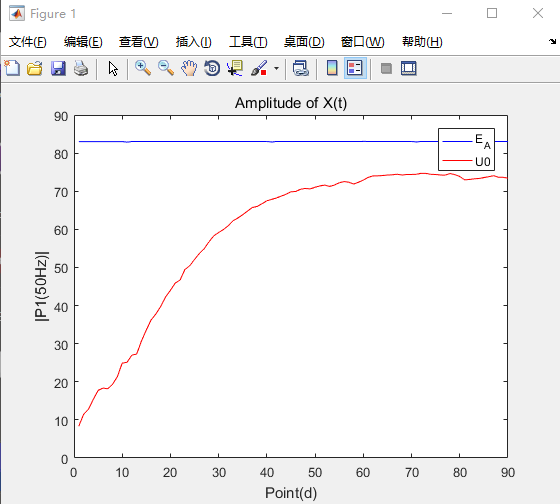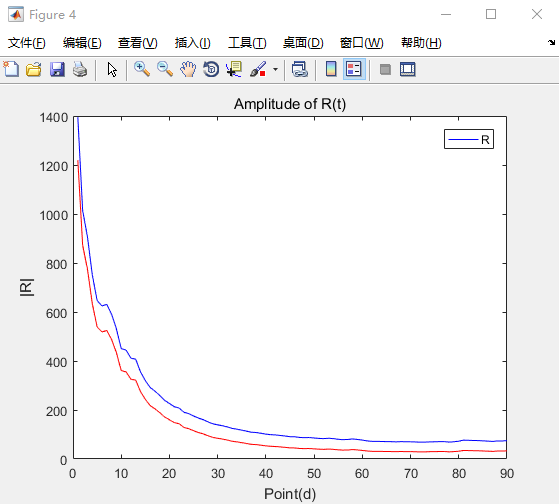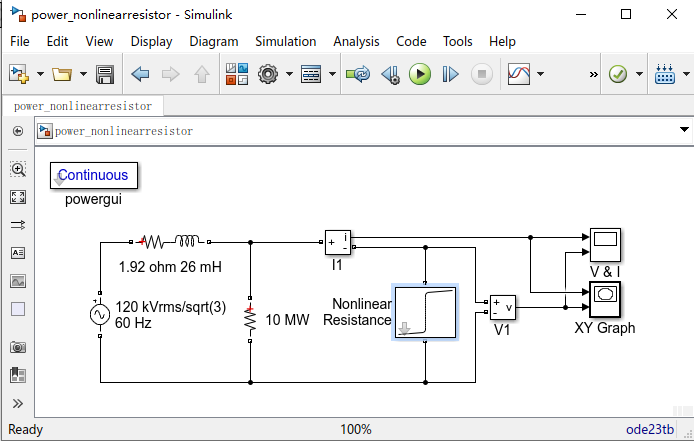### 1.1 供电电压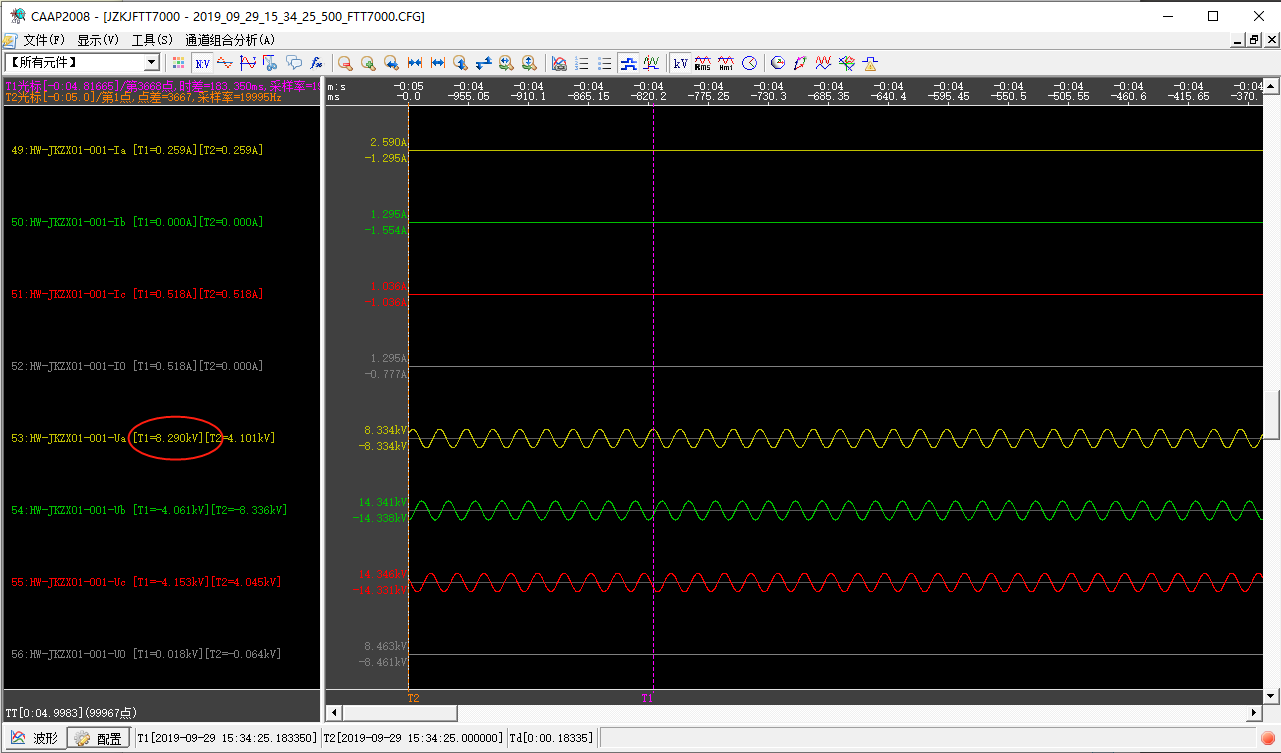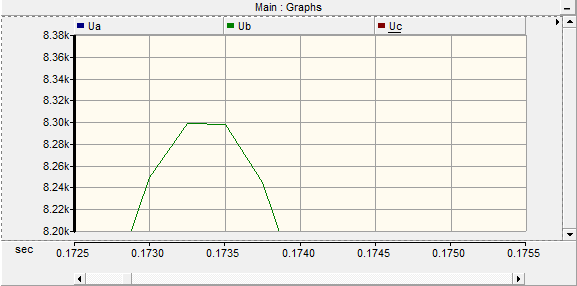### 1.2 设置变压器及开关站端口电容。

2条馈线发生单相接地故障后，本身在空载状态容流非常小，可以认为测定到的全部为背景容流，有效值为4.072A。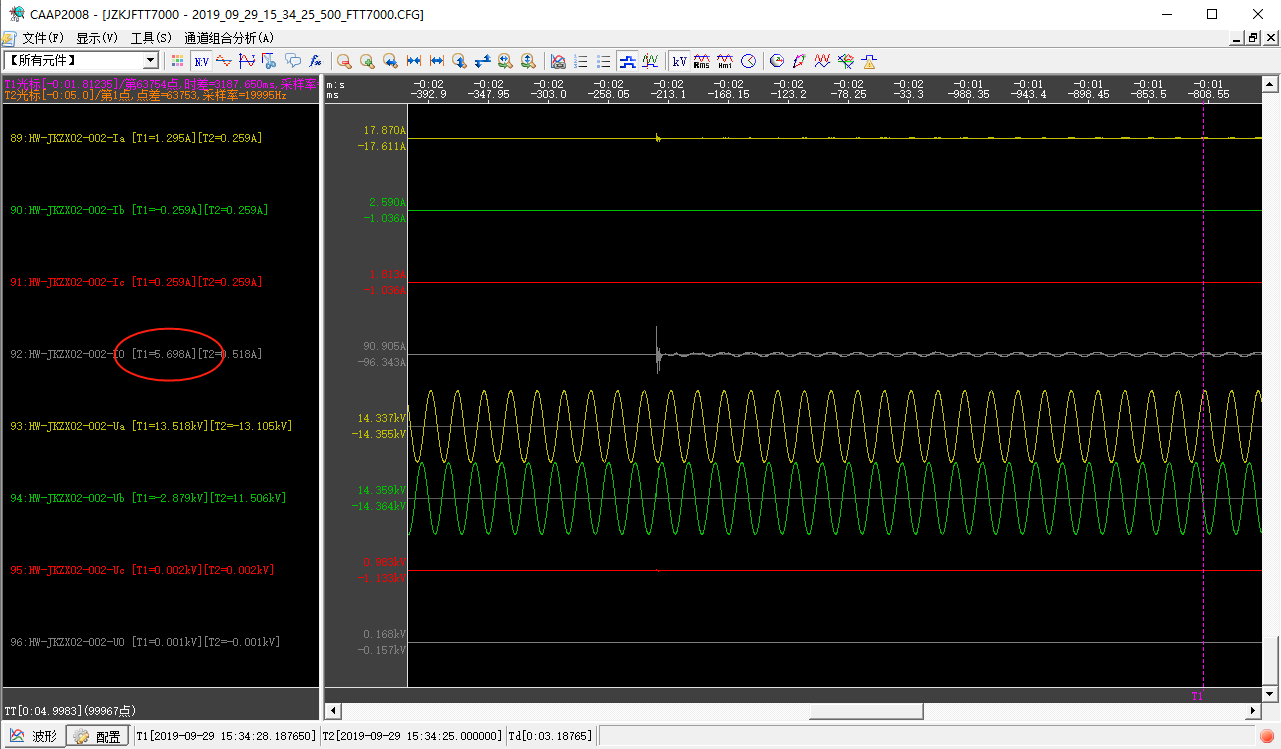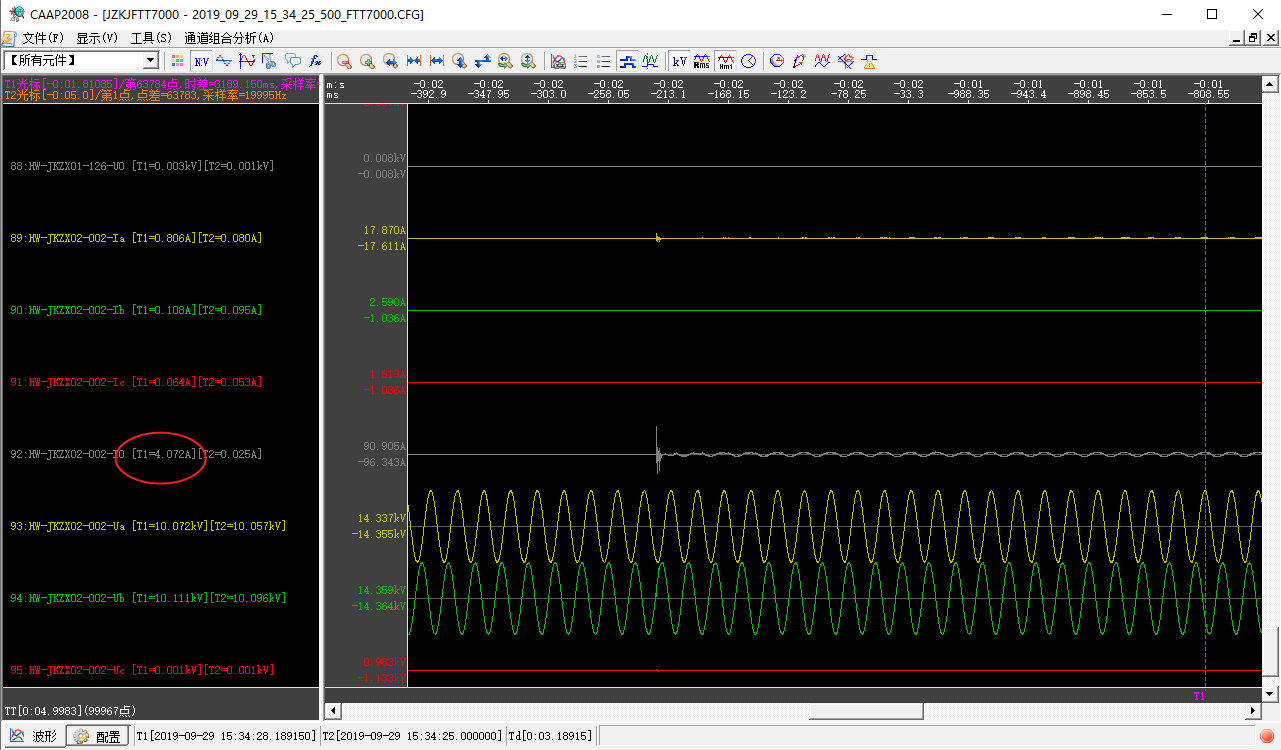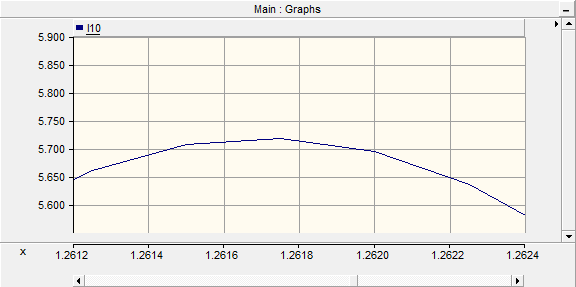### 1.3 考核场地网电阻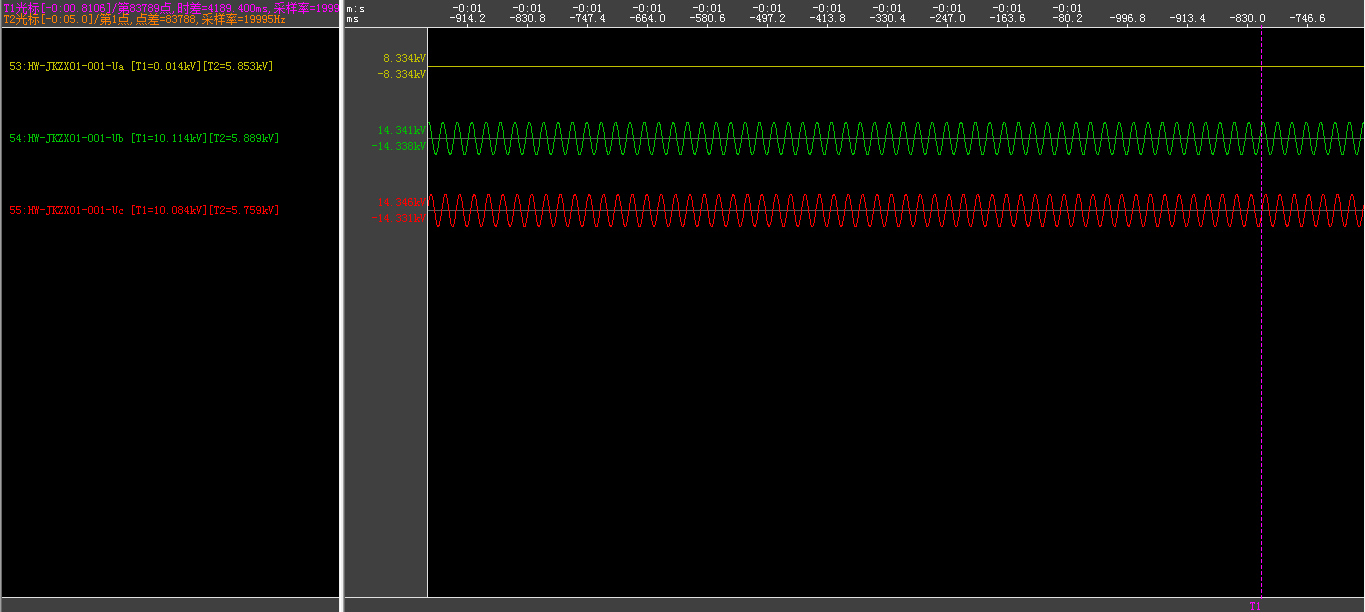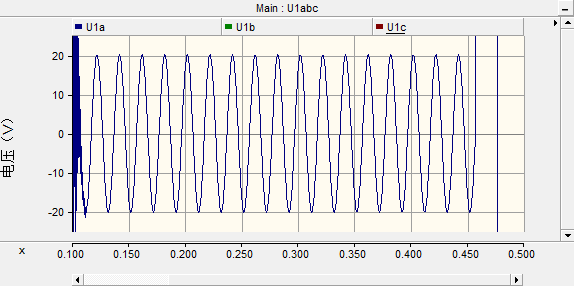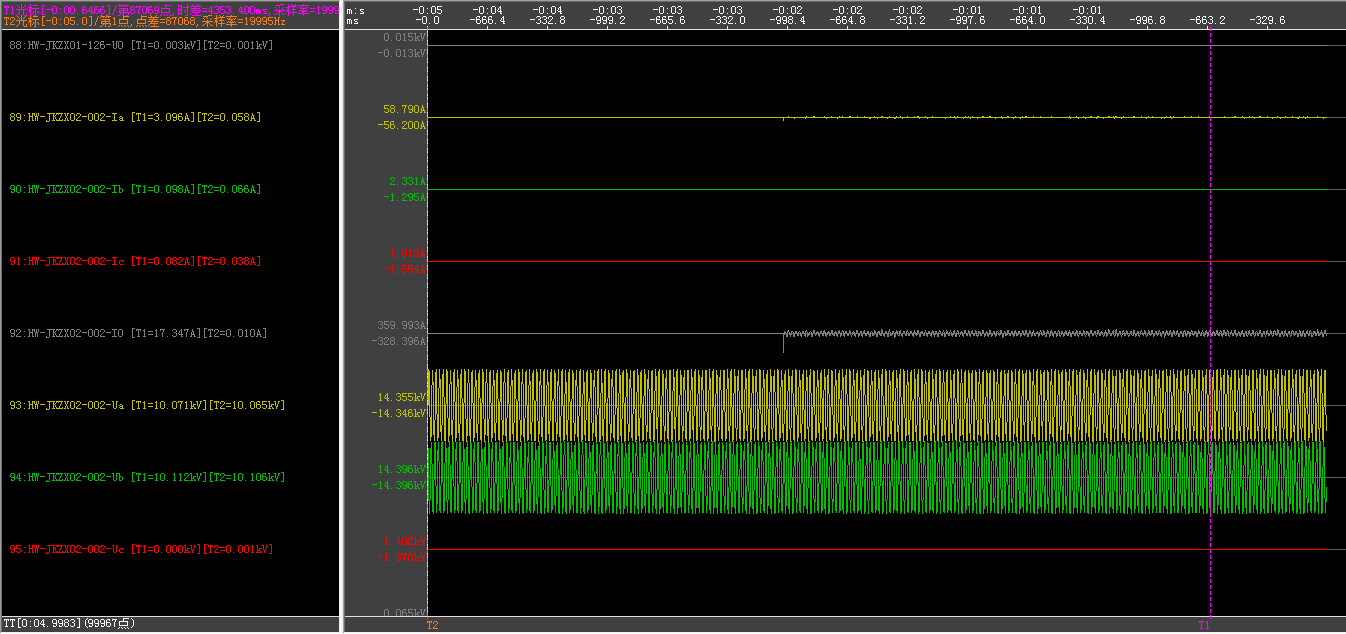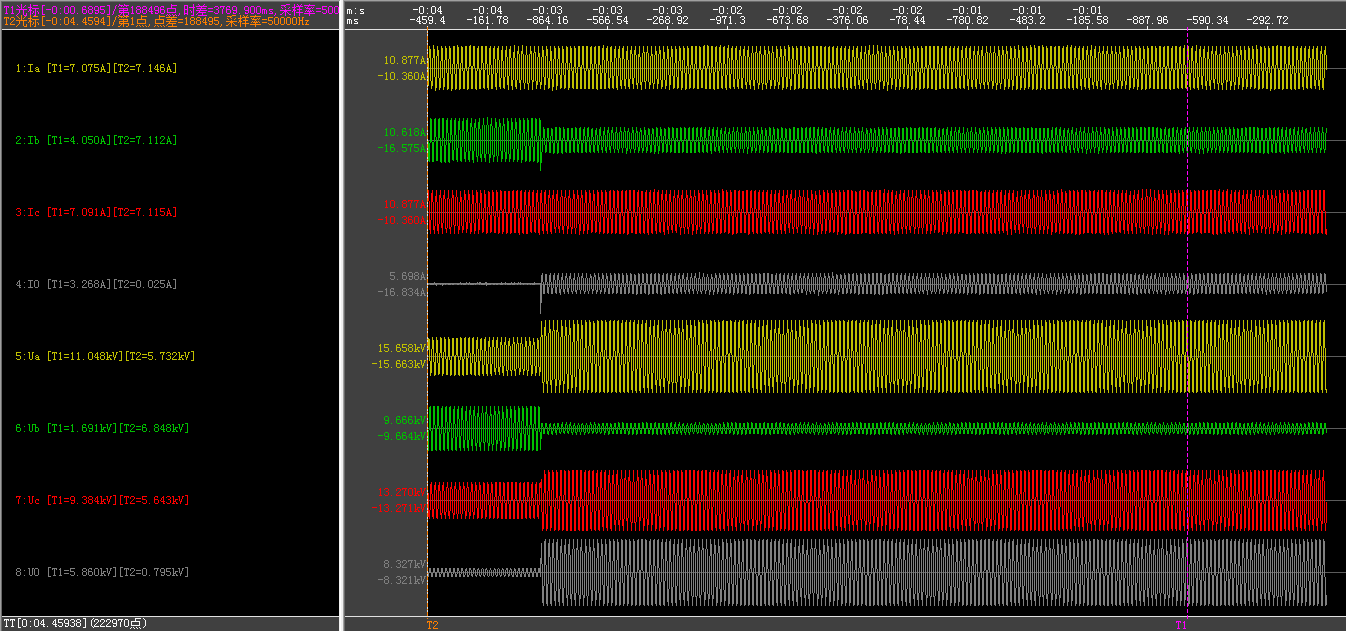$R=1691(V)/ 3.268(A）=517.44（Ω）$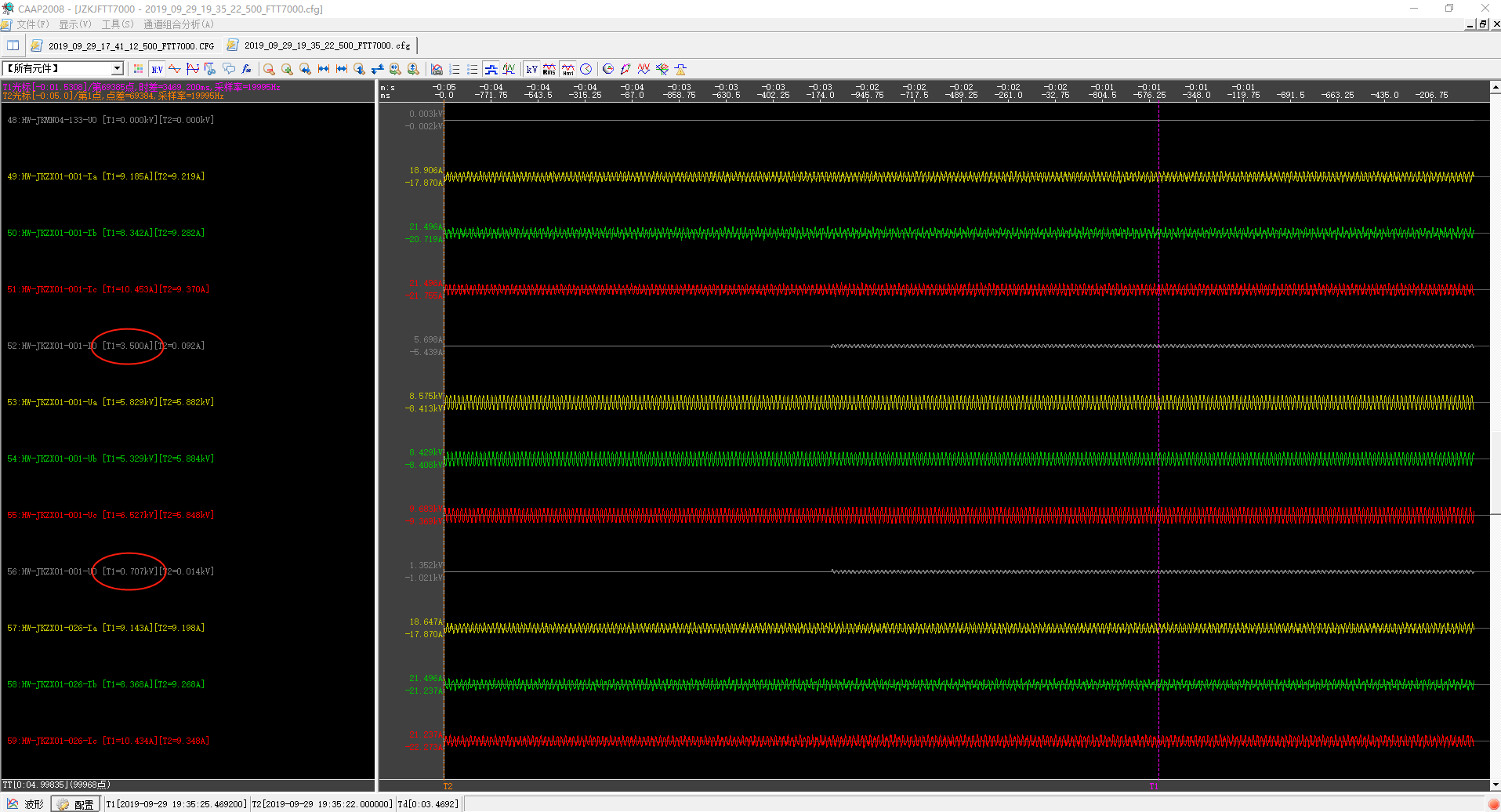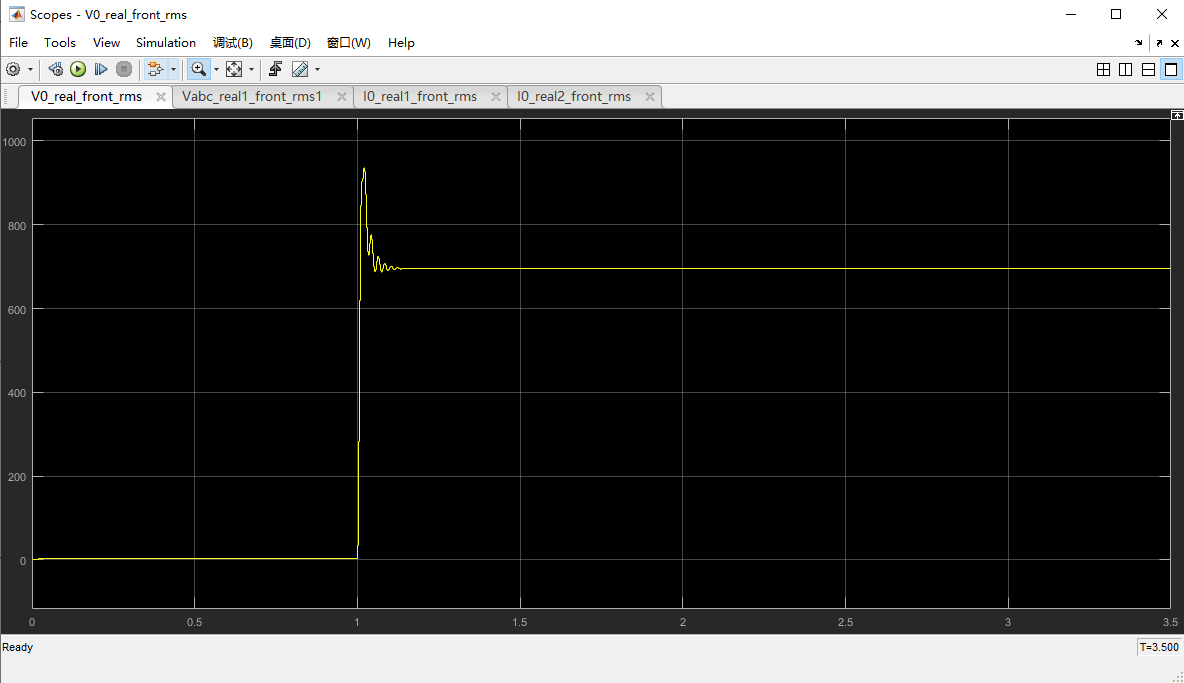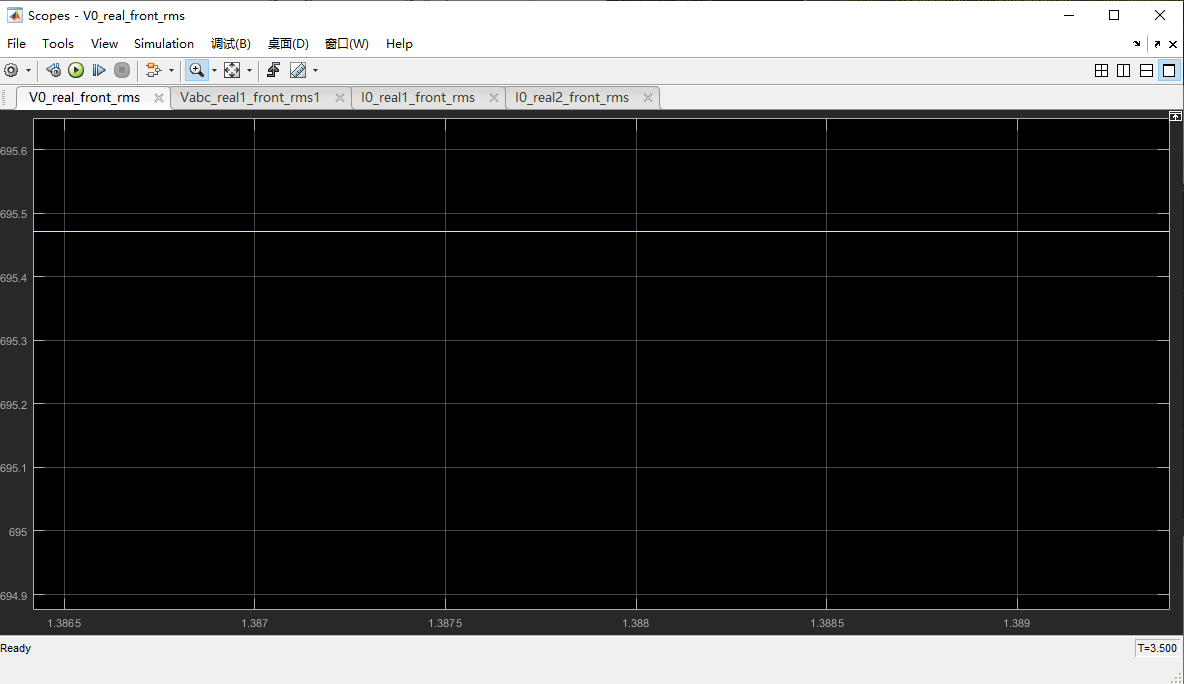707V（实测）对应700V（仿真）；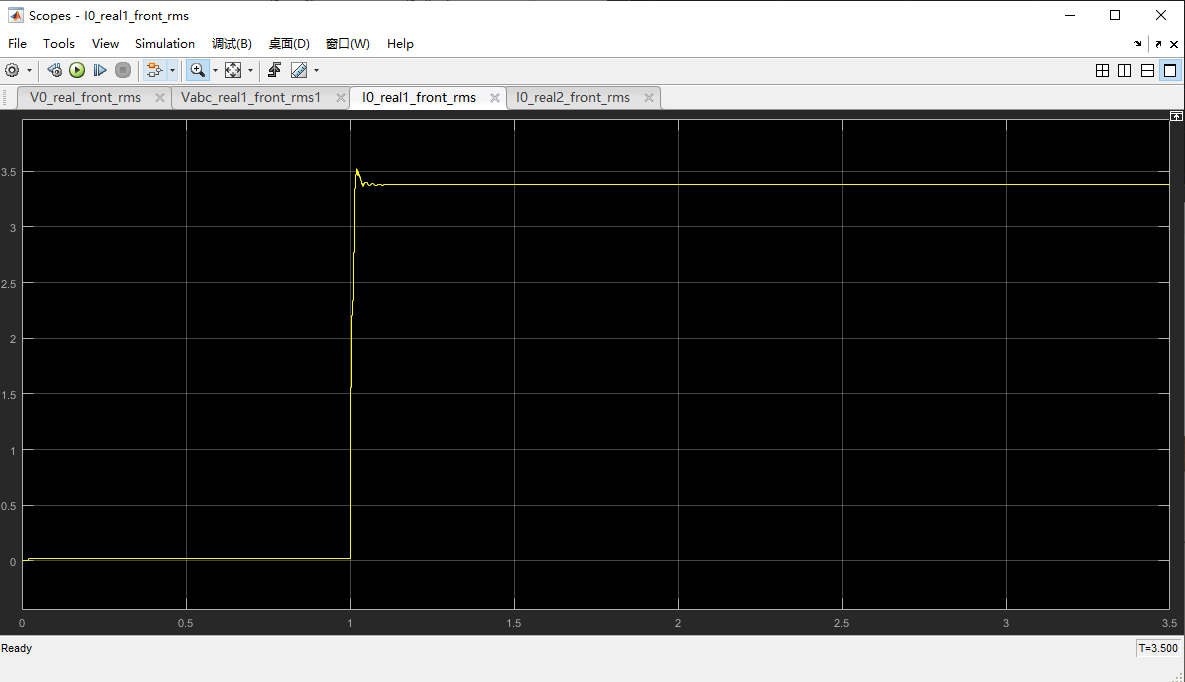3.5A（实测）对应3.38A（仿真）。

## 2. 考虑对地导纳的中性点电压数学模型

### 2.1 数学模型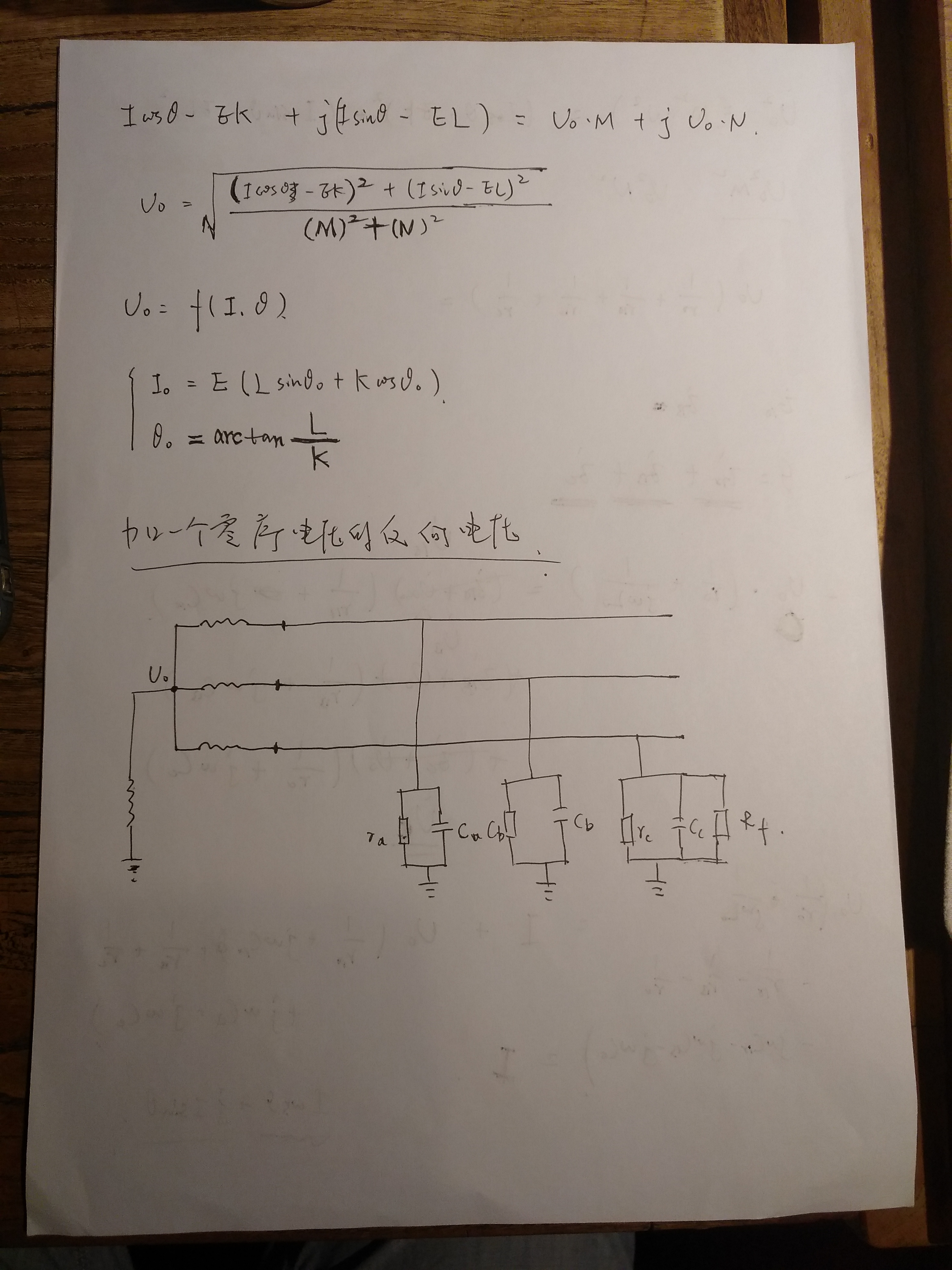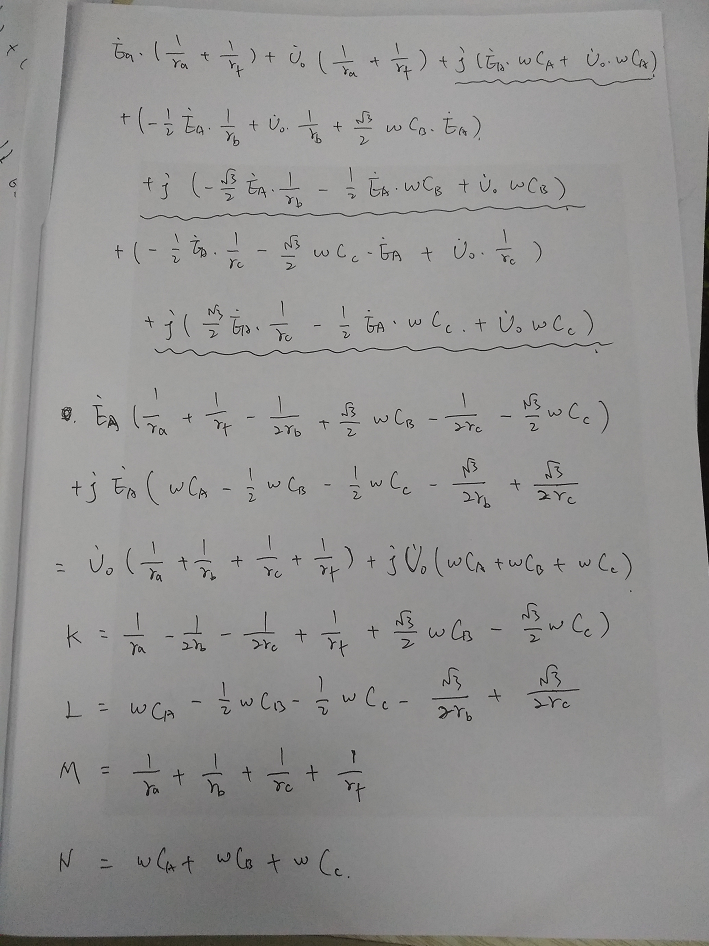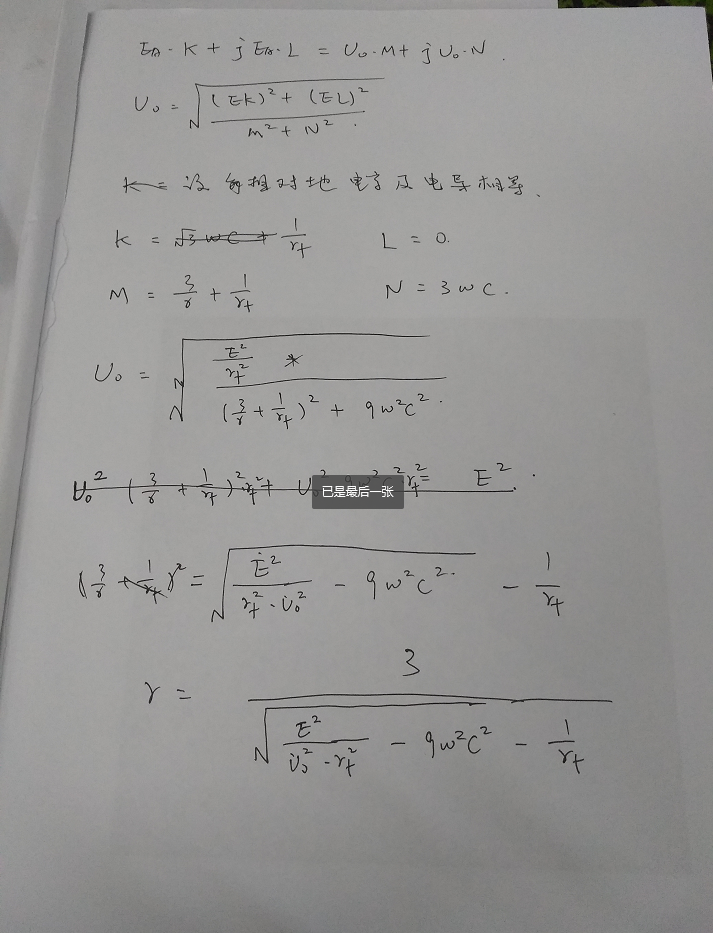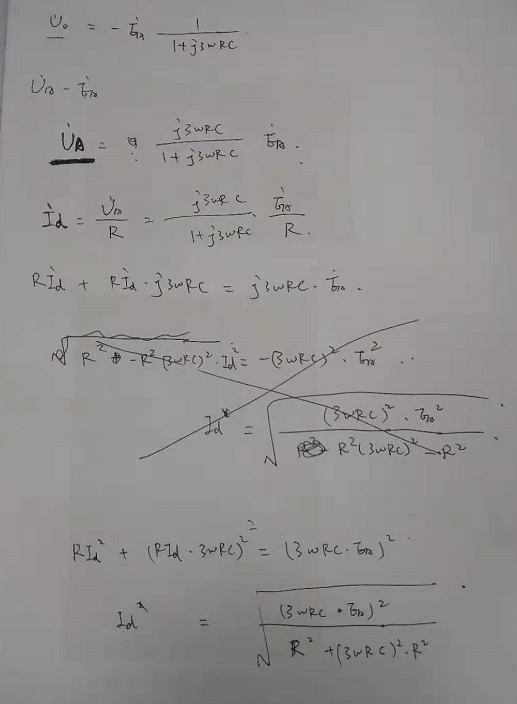### 2.2 数据分析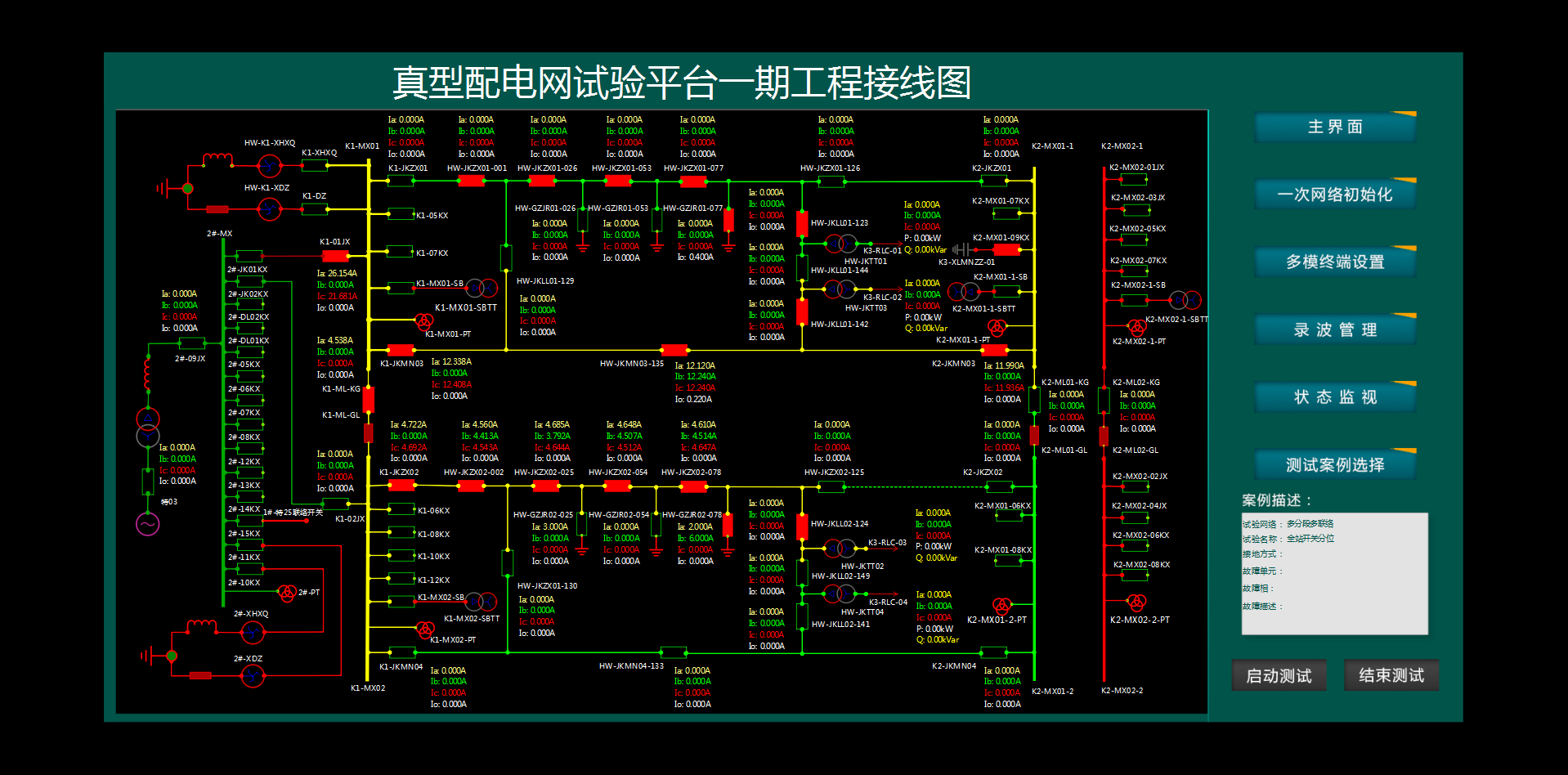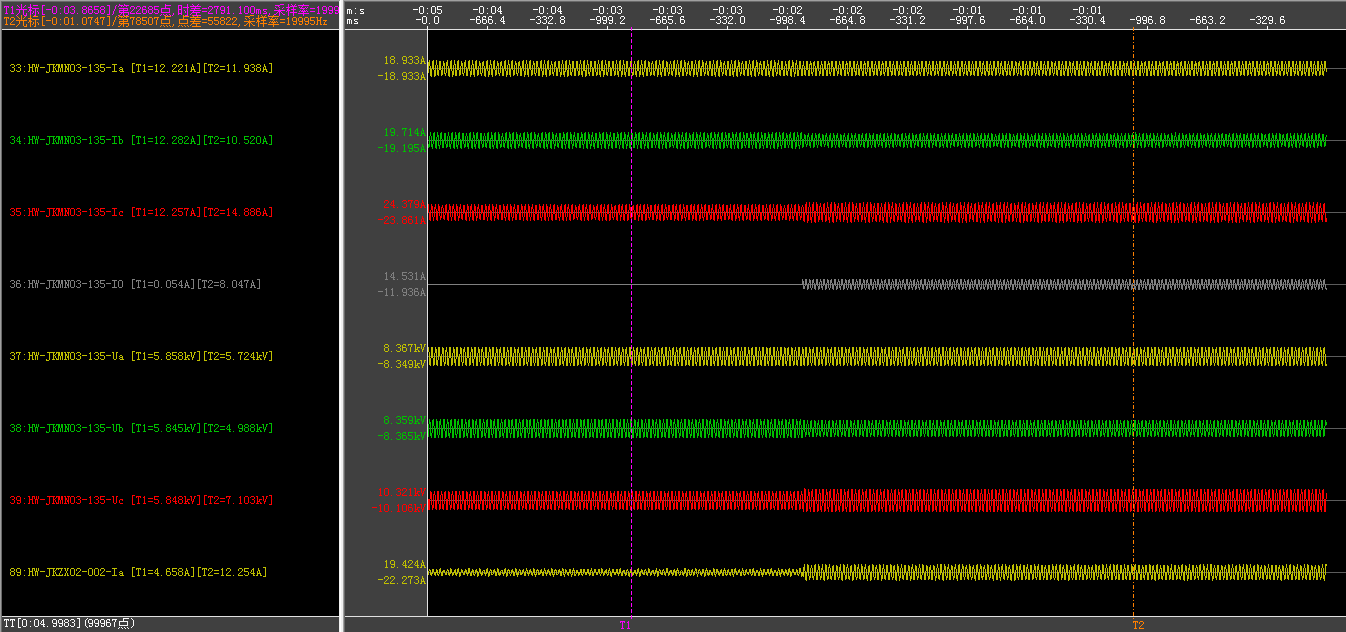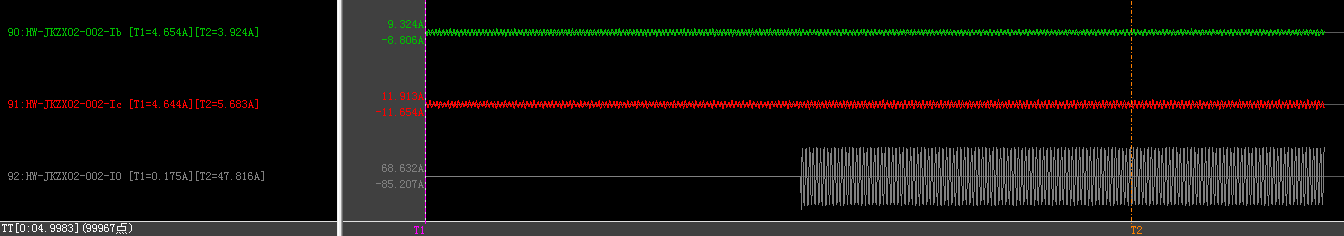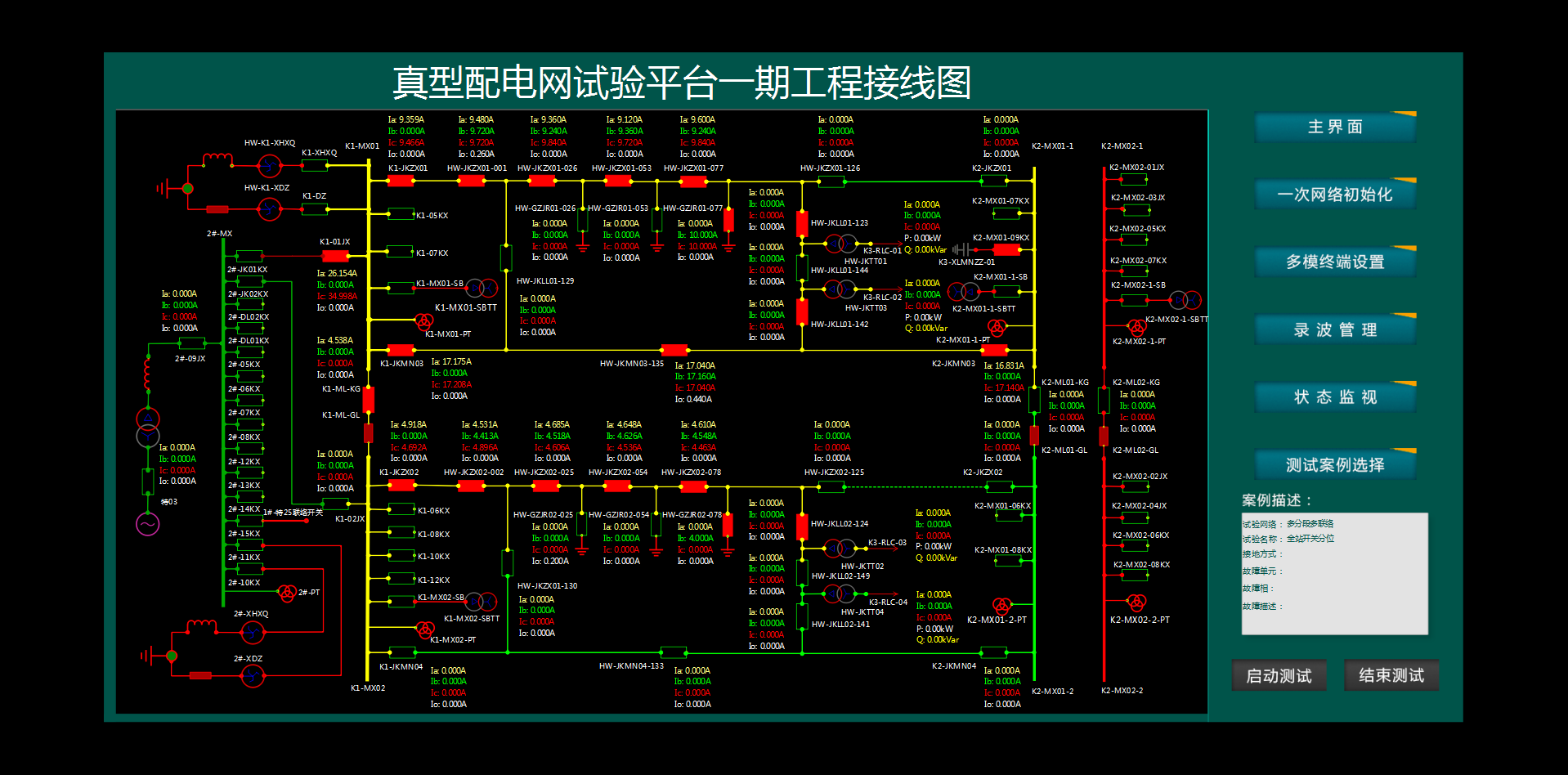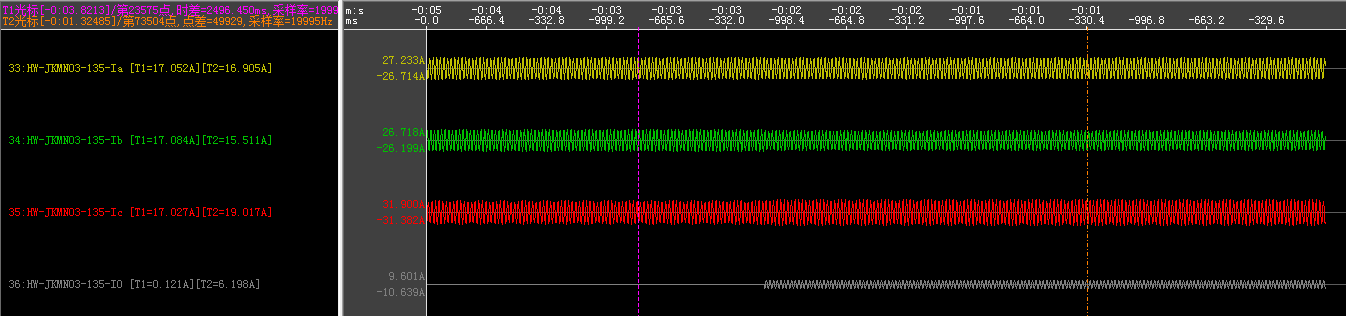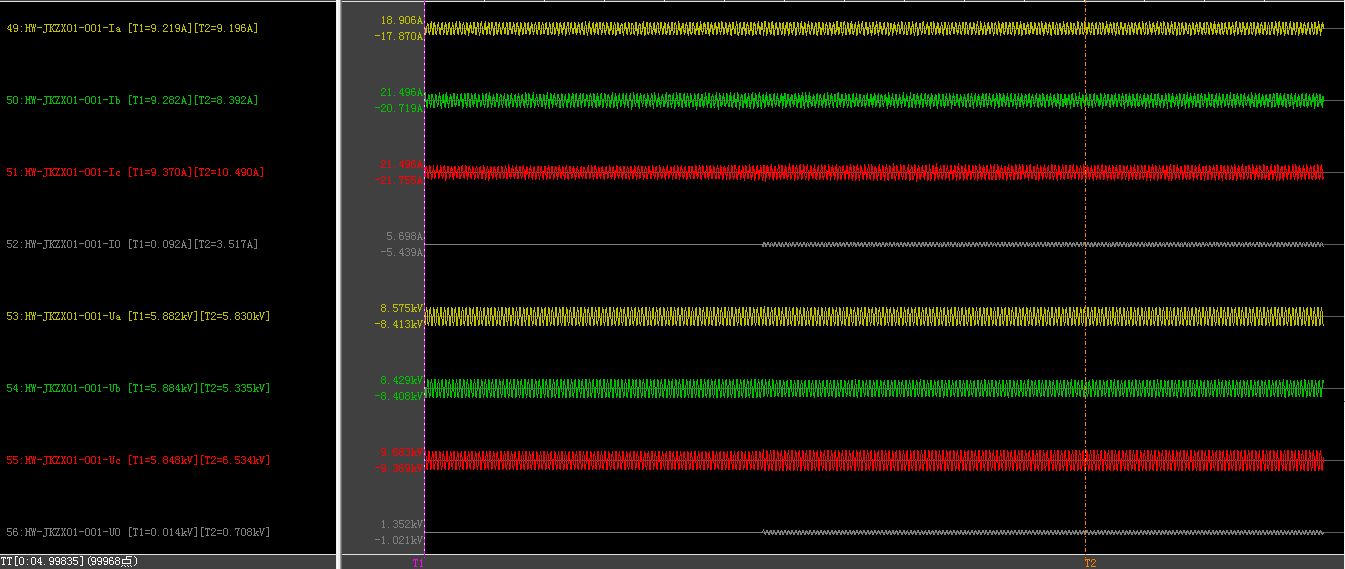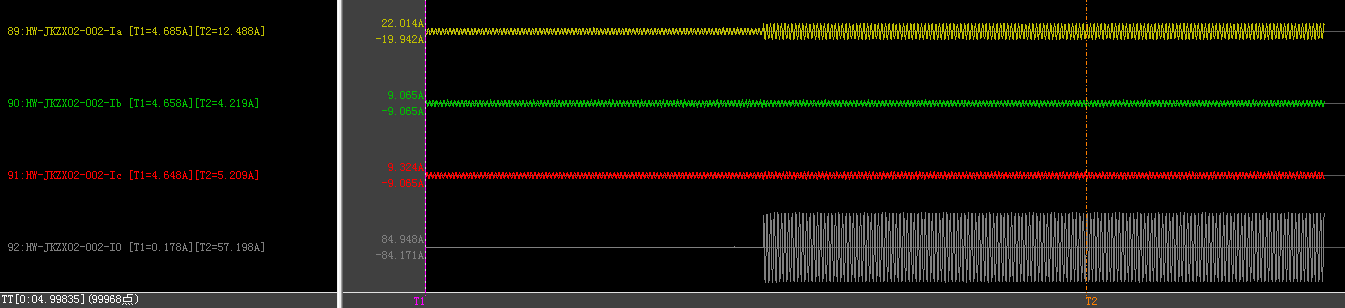$U_A$ $U_B$ $U_C$ $U_0$ $U_A$ $U_B$ $U_C$ $U_0$
5884 5882 5851 14 5830 5335 6534 708
$I_{1A}$ $I_{1B}$ $I_{1C}$ $I_{10}$ $I_{1A}$ $I_{1B}$ $I_{1C}$ $I_{10}$
9.255 9.281 9.378 0.086 9.196 8.392 10.49 3.517
$I_{2A}$ $I_{2B}$ $I_{2C}$ $I_{20}$ $I_{2A}$ $I_{2B}$ $I_{2C}$ $I_{20}$
4.694 4.67 4.654 0.187 12.488 4.219 5.209 57.198
$I_{3A}$ $I_{3B}$ $I_{3C}$ $I_{30}$ $I_{3A}$ $I_{3B}$ $I_{3C}$ $I_{30}$
17.079 17.086 17.035 0.119 16.905 15.511 19.017 6.198

#### 2.2.1 计算线路对地电容

$r=\frac{3}{\sqrt{\frac{E^{2}}{U_{0}^{2} \cdot r_{f}^{2}}-9 \omega^{2} C^{2}}-\frac{1}{r_{f}}}$

#### 2.2.2 计算接地阻抗公式

$\left(9+9 \omega^{2} c^{2} r^{2}\right) r_{f}^{2}+6 r \cdot r_{f}+r^{2}+\frac{E^{2}}{U_{0}^{2}}=0$

$\left(1-\omega^{2} c^{2} r^{2}\right) \frac{E^{2}}{U_{0}^{2}}-\omega^{2} c^{2} r^{2} \cdot r^{2} \geqslant 0$

#### 2.2.3 找另一组电容量的进行计算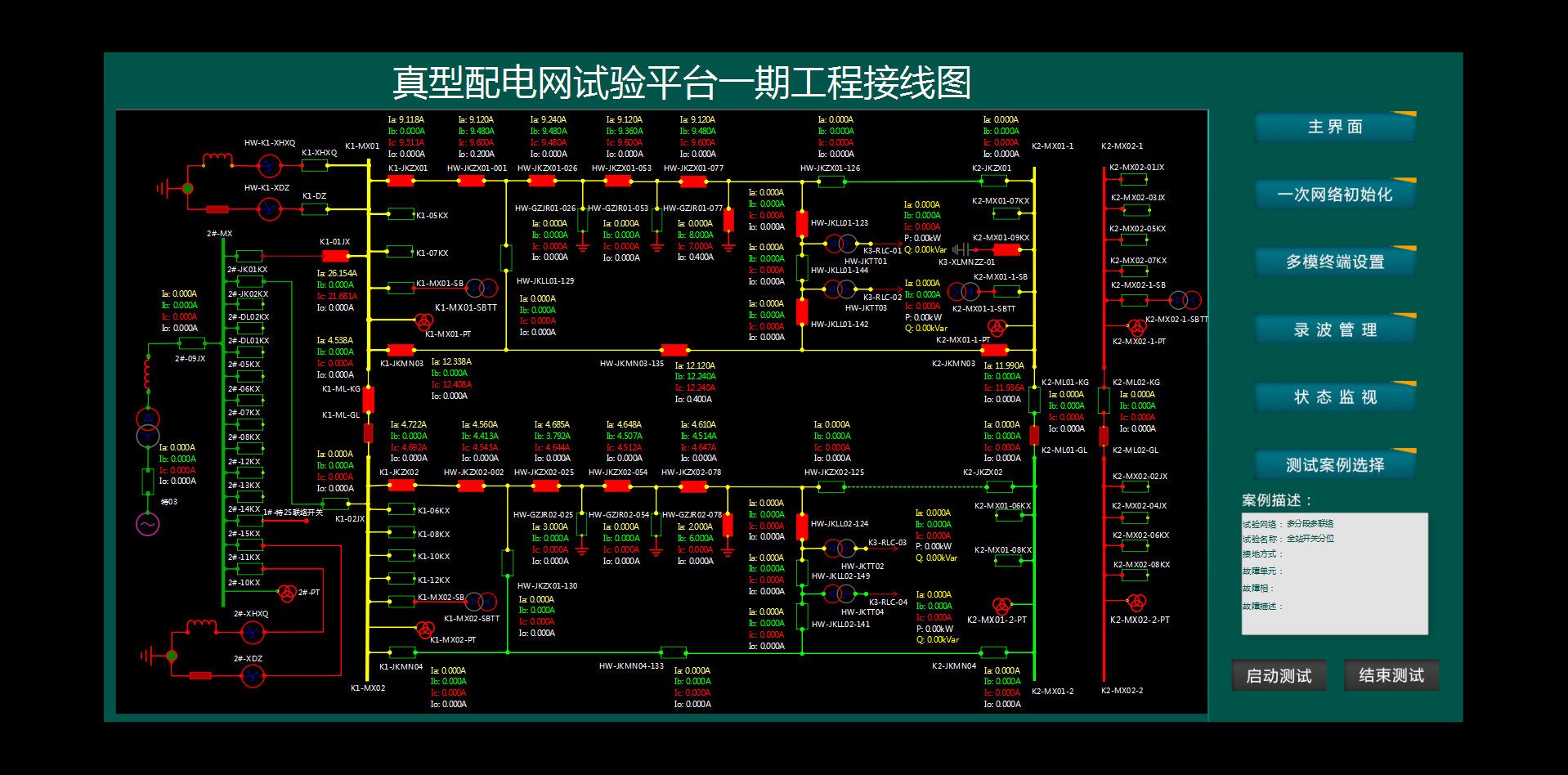1、2、3馈线带3+4档电容器。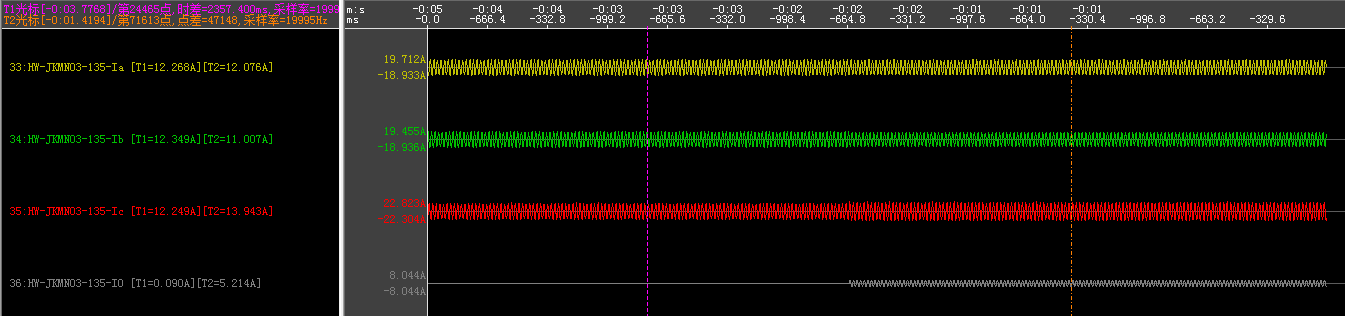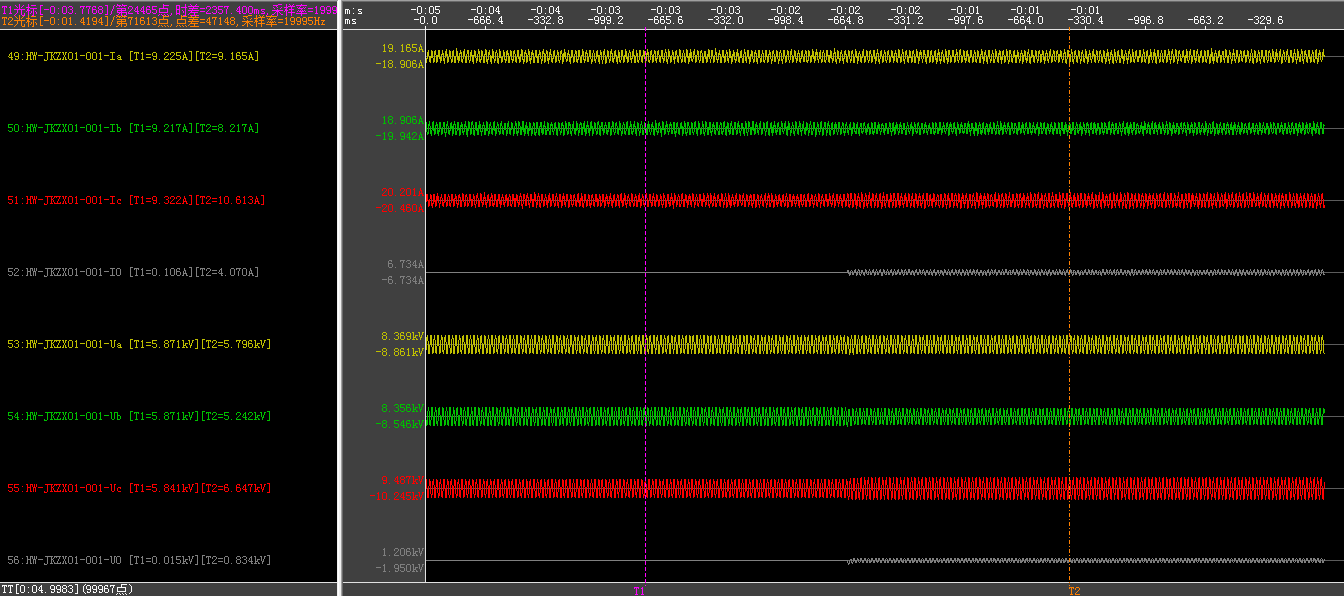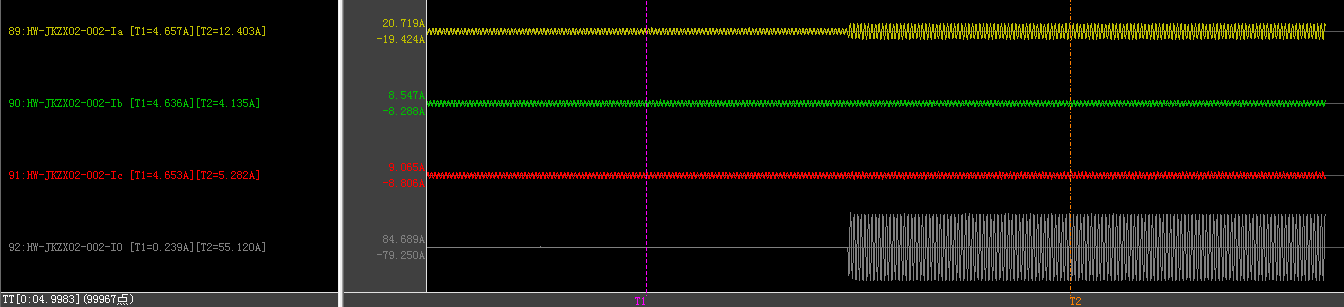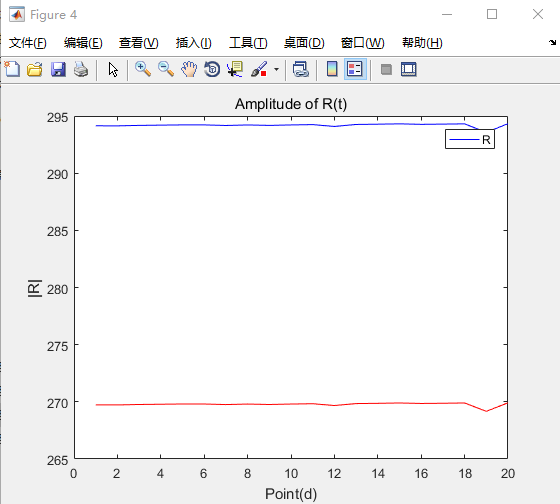#### 2.2.4 6档电容，和计算出940欧姆电阻组只有电容器组别不同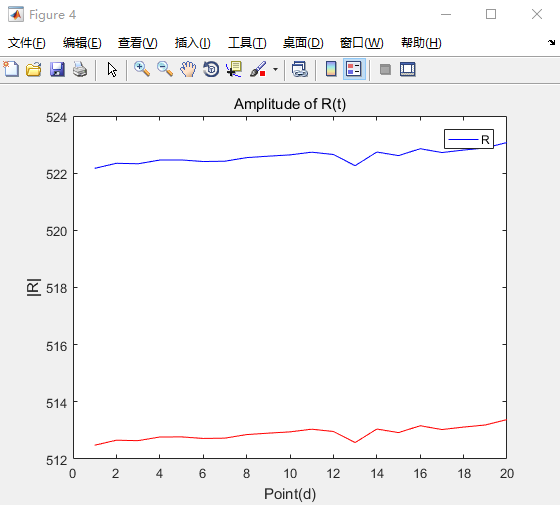##### 2.2.4.7 1000欧姆电阻，只带线路电容器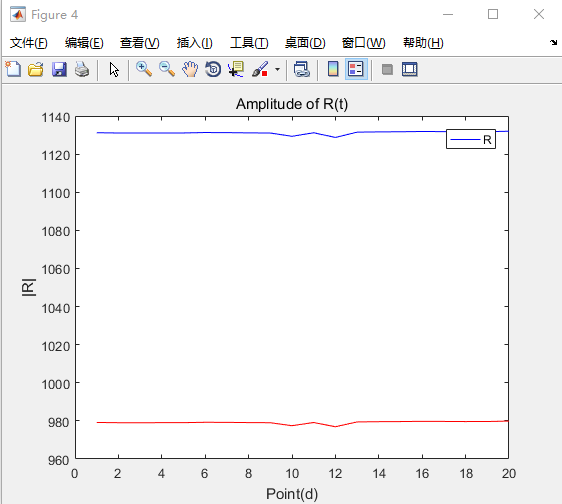### 2.3 电弧的辨识